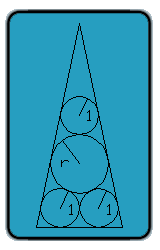All about flooble | fun stuff | Get a free chatterbox | Free JavaScript | Avatarsperplexus dot infoFour Tangent Circles (Posted on 2003-10-20)Four circles are tangent to each other and to a triangle that surrounds them, as shown in the figure. Each of the three circles in the corners have a radius of 1 cm. What is the radius of the circle in the middle?No Solution Yet Submitted by Bryan Rating: 4.1429 (7 votes)Comments: ( Back to comment list | You must be logged in to post comments.)Solution| Comment 7 of 13 |If we divide the isoceles triangle in two right triangles we get a triangular relation based on the top angle (I called theta). Sin(theta)=1/(1+L1)=r/(r+2+2/(r-1)). This gives us L1=2/(r-1).
Then we use the right triangle from the center of the circle with radius r, to the center of one of the circles of the botom and the quadrant between circles of radius 1, this gives us the relation L2=√(r*(r+2))-r. Finally using the first triangular relation, we get the transient equation:(r-1)*(cos(theta)+1)=cos(theta)*((r-1)*(tan(theta)+√(r*(r+2))+r+2)+2) where theta = arcsin(r*(r-1)/(2+(r-1)*(r+2))). Solving the mentioned equation, the radius of the circle in the middle is r=1.51067547264cm
 Posted by Antonio on 2003-12-02 01:11:36Please log in:

 Search: Search body:
Forums (0)